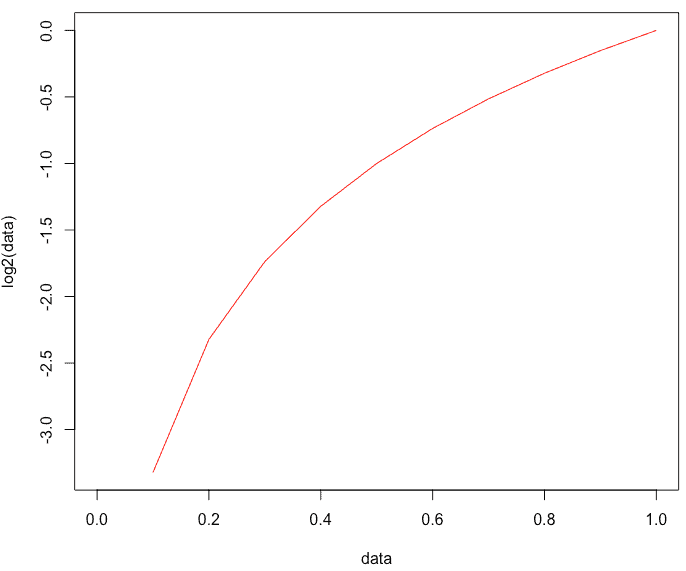# log2 in R: How to Use log2() Function in R Language

The log() function computes logarithms, by default, it calculates the natural logarithms. The log10() function computes common (for example, base 10) logarithms. The log2() function computes binary (i.e., base 2) logarithms. The common log(x, base) function computes logarithms with base.

## log2 in R

The log2() is an inbuilt R mathematical function that calculates the binary (base 2) logarithm. The log() function calculates natural logarithms by default. The log2() method computes the logarithm of the given column in base 2.

### Syntax

``log2(x)``

### Parameters

It takes x as a column to calculate.

### Example

Let’s find the log2 of 11 using the log2() function.

``log2(11)``

#### Output

`` 3.459432``

Let’s find the log2 value of 0 and 1.

``````log2(0)
log2(1)``````

#### Output

`````` 0
 -Inf``````

## Plot the log2() function in R

The most used plotting function in R programming is the plot() function. It is an inbuilt generic function that has many methods called according to the type of object passed to the plot().

Open the rstudio and write the following code.

``````data <- seq(0, 1, by = 0.1)
plot(data, log2(data), typ = "l", col = "red")``````

#### Output## Find the log2() of vector

To find the log2 of vector, pass the vector to the log2() function. Before that, you have to create a vector sequence if it is not available to you. Let’s create a vector sequence for our example.

``````vc <- (rep(1:5))
cat("The vector sequence is: ")
cat(vc, "\n")``````

#### Output

``The vector sequence is: 1 2 3 4 5``

To find the log2 of this vector, pass the sequence to a log2() function.

``````vc <- (rep(1:5))
cat("The vector sequence is: ")
cat(vc, "\n")
cat("Find the log2() of vector sequence is: ")
cat(log2(vc))``````

#### Output

``````The vector sequence is: 1 2 3 4 5
Find the log2() of vector sequence is: 0 1 1.584963 2 2.321928``````

That is it for log2() function in R language.

Categories R